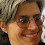## 2016-04-11

In my development algebra classes, I push radicals further forward, closer to the start of the semester than most other instructors or textbooks. I want them to be discussed jointly with exponents, so we can really highlight the inverse relation with exponents, and that knowledge of the rules of one is effectively equivalent knowledge of the other. Also: Based on the statistics I keep, success on the exponents/radicals test is the single best predictor of success on the comprehensive, university-wide final exam.

There are, of course, many errors made by students learning to read and write radicals for effectively the first time. Here's an exceedingly common category, to write something like ($$x > 0$$):
$$\sqrt{16} = 4 = 2$$
$$\sqrt{x^8} = x^4 = x^2 = x$$

Any of these expressions may or may not have a radical written over them (including, e.g., $$\sqrt{4} = \sqrt{2}$$). That is: Students see something "magical" about radicals, and sometimes keep square-rooting any expression in sight, until they can no longer do so. This is common enough that I have few interventions in my mental toolbox ready for when this occurs in any class:
1. Go to the board and, jointly with the whole class, start asking some true-or-false questions. "T/F: $$\sqrt{4} = \sqrt{2}$$ ← False. $$\sqrt{4} = 2$$ ← True." Briefly discuss the difference, and the location of $$\sqrt{2}$$ on the number line. Emphasize: Every written symbol in math makes a difference (any difference in the writing, and it has a different meaning).

2. Prompt for the following on the board. "Simplify: $$3 + 5 = 8$$." Now ask: "Where did the plus sign go? Why are you not writing it in the simplified expression? Because: You did the operation, and therefore the operational symbol goes away. The same will happen with radicals: If you can actually compute a radical, then the symbol goes away at that time."

That's old hat, and those are techniques I've been using for a few years now. The one new thing I noticed last night (as I write this) is that there is actually something unique about the notation for radicals: Of the six basic arithmetic operations (add, subtract, multiply, divide, exponents, radicals), radicals are the only binary operation where one of the two parameters may not be written. That is, for the specific case of square roots, there is a "default" setting where the index of 2 doesn't get written -- and there's no analogous case of any other basic operator being written without a pair of numbers to go with it.

I wonder if this contributes to the apparent "magical" qualities of radicals (specifically: students pay more attention to the visible numbers, whereas I am constantly haranguing students to look more closely at the operators in the writing)? Hypothetically, if we always wrote the index of "2" visibly for square roots (as for all other binary operators), would this be more transparent to students that the operator only gets applied once (at which point radical and index simplify out of the writing)? And perhaps this would clear up a related problem: students occasionally writing a reduction as a new index, instead of a factor (e.g., $$\sqrt{18} = \sqrt{9 \times 2} = \sqrt{2}$$)?

That would be a pretty feasible experiment to run in parallel classes, although it would involve using nonstandard notation to make it happen (i.e., having students explicate the index of "2" for square roots all the time). Should we consider that experiment?

1.In the operation 0-x, 0 may be omitted. Or else you could consider the square root a unary operation.

1.But the expression -x would usually not be defined as a subtraction.

Regardless of how we interpret square roots, it's a notational outlier, it's confusing to students, and it clashes with the notation for higher roots and all other elementary operations (especially including its inverse, the squaring function).

2.I don't see the distinction. If we interpret negation and subtraction as completely different operations, even though they use the same symbol and negation is syntactically identical to subtraction with an omitted zero, then why can't we do the same with radicals?

3.Here's the distinction, which I overly abbreviated in the main blog: the radical can still be simplified. That is: while -x is the simplest way to write that expression, √x is usually not (e.g., in the cases of √9 or √45), and it's the only apparently unary operator where that's the case. In other words: from the students' perspective, the radical is the only basic operator where you can "do something" to it (simplify it) without any 2nd parameter in sight (due to the default index of 2).

Thanks for helping me to clarify that point.

2.It's worth trying. And I'll be interested in your results. I often talk about the "invisible two" sitting there. (As I talk about the "invisible 1" as the co-efficient of a variable sometimes.)

1.Thanks for the vote of support!

3.I think you ought to try it. You'll run into some friction because none of the textbooks will have the "implicit 2" written in, but it would probably be a good re-inforcement for the students to explicitly write the 2 for all their assignments. That is, whenever they see the radical without the 2, they should transcribe it in their homework with the 2.

1.That's a great point, to avoid rewriting all the book exercises.